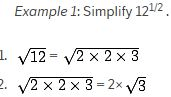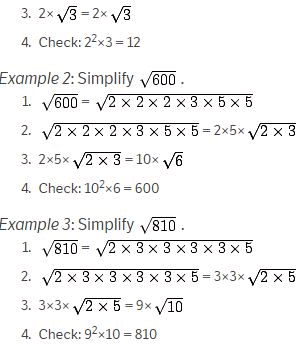UPSC  >  Introduction: Simplification & Approximation

# Introduction: Simplification & Approximation - Notes | Study UPSC Prelims Paper 2 CSAT - Quant, Verbal & Decision Making - UPSC

 Table of contentsIntroductionBasic Rules of SimplificationTips to Crack Approximation1 Crore+ students have signed up on EduRev. Have you?

Introduction

• The Quantitative Aptitude section of the different competitive examinations consists of questions such as Simplification, Number Series, Permutation & Combination, Quadratic Equation, Data Interpretation, Data Analysis and other Miscellaneous questions.
• There are almost 10 to 12 questions in the paper which deal with topics such as Percentage and Average, ratio & proportions, partnerships, Profit & loss, calculations of simple & compound interests etc.

Here is a short study guide to help you crack questions on “Simplification and Approximation

Basic Rules of Simplification

#### BODMAS Rule

• It defines the correct sequence in which operations are to be performed in a given mathematical expression to find the correct value.
• This means that to simplify an expression, the following order must be followed -

B = Bracket,
O = Order (Powers, Square Roots, etc.)
D = Division

M = Multiplication

S = Subtraction

• Hence, to solve simplification questions correctly, you must apply the operations of brackets first.
• Further, in solving for brackets, the order - (), {} and [] - should be strictly followed.
• Next, you should evaluate exponents (for instance powers, roots etc.)
• Next, you should perform division and multiplication, working from left to right. (division and multiplication rank equally and are done left to right).
• Finally, you should perform addition and subtraction, working from left to right. (addition and subtraction rank equally and are done left to right).

Example 1:  Solve 12 + 22 ÷ 11 × (18 ÷ 3)^2 - 10

Solution:

= 12 + 22 ÷ 11 × 6^2 - 10 (Brackets first)
= 12 + 22 ÷ 11 × 36 - 10 (Exponents)
= 12 + 2 × 36 - 10 = 12 + 72 - 10 (Division and multiplication, left to right)
= 84 - 10 = 74 (Addition and Subtraction, left to right)

Example 2:  Solve 4 + 10 - 3 × 6 / 3 + 4

Solution:

= 4 + 10 - 18/3 + 4 = 4 + 10 - 6 + 4 (Division and multiplication, left to right)
= 14 - 6 + 4 = 8 + 4 = 12 (Addition and Subtraction, left to right)

To Solve Modulus of a Real Number

• The Modulus (or the absolute value) of x is always either positive or zero, but never negative.
• For any real number x, the absolute value or modulus of x is denoted by |x| and is defined as

|x|= x {if x ≥ 0} and −x {if x < 0}

Exmaple: Solve |8|

Solution: |8| = |-8|  = 8

Tips to Crack Approximation

Conversion of decimal numbers to nearest number

• To solve such questions, first convert the decimal to the nearest value.
• Then simplify the given equation using the new values that you have obtained.

Example1:  Solve 4433.764 - 2211.993 - 1133.667 + 3377.442

Solution: Here,
4433.764 = 4434
2211.993 = 2212
1133.667 = 1134
3377.442 = 3377

Now simplify, 4434 -  2212 - 1134 + 3377 = 4466

Example 2: Solve 530 x 20.3% + 225 x 16.8%

Solution: Here, 20.3% becomes 20% and 16.8% becomes 17%
Now, simplify 530 x 20% + 225 x 17%
= 106 + 38.25 = 144.25

Approximation of Square Roots

• To simplify a square root, you can follow these steps:
• Factor the number inside the square root sign.
• If a factor appears twice, cross out both and write the factor one time to the left of the square root sign. If the factor appears three times, cross out two of the factors and write the factor outside the sign, and leave the third factor inside the sign. Note: If a factor appears 4, 6, 8, etc. times, this counts as 2, 3, and 4 pairs, respectively.
• Multiply the numbers outside the sign.
• Multiply the numbers left inside the sign.
• To simplify the square root of a fraction, simplify the numerator and simplify the denominator.

NOTE: Check that the outside number squared times the inside number should equal the original number inside the square root.Following are some solved examples to help you prepare well for the upcoming exams:

Ques1. A man has Rs. 480 in the denominations of one-rupee notes, five-rupee notes, and ten-rupee notes. The number of notes of each denomination is equal. What is the total number of notes that he has?
A.    45
B.    60
C.    75
D.    90

Explanation:

Let the number of notes of each denomination be x.

Then x + 5x + 10x = 480

=> 16x = 480

Therefore, x = 30.

Hence, total number of notes = 3x = 90.

Ques2. There are two examinations rooms A and B. If 10 students are sent from A to B, then the number of students in each room is the same. If 20 candidates are sent from B to A, then the number of students in A is double the number of students in B. The number of students in room A is:
A.    20
B.    80
C.    100
D.    200

Explanation:

Let the number of students in rooms A and B be x and y respectively.

Then, x - 10 = y + 10      x - y = 20 .... (i)

and x + 20 = 2(y - 20)      x - 2y = -60 .... (ii)

Solving (i) and (ii) we get: x = 100 , y = 80.

Therefore, The required answer A = 100.

Ques3. The price of 10 chairs is equal to that of 4 tables. The price of 15 chairs and 2 tables together is Rs. 4000. The total price of 12 chairs and 3 tables is:
A.    Rs. 3500
B.    Rs. 3750
C.    Rs. 3840
D.    Rs. 3900

Explanation:

Let the cost of a chair and that of a table be Rs. x and Rs. y respectively.

Then, 10x = 4y   or   y =    5/2 x.
Therefore, 15x + 2y = 4000

=> 15x + 2*(5/2)x = 4000

=> 20x = 4000

Therefore, x = 200.

So, y = (5/2) * 200 = 500.

Hence, the cost of 12 chairs and 3 tables = 12x + 3y

= Rs. (2400 + 1500)

= Rs. 3900.

Ques4. If a - b = 3 and a2 + b2 = 29, find the value of ab.
A.    10
B.    12
C.    15
D.    18

Explanation:

2ab = (a2 + b2) - (a - b)2

= 29 - 9 = 20

=> ab = 10.

Ques5. The price of 2 sarees and 4 shirts is Rs. 1600. With the same money one can buy 1 saree and 6 shirts. If one wants to buy 12 shirts, how much shall he have to pay ?
A.    Rs. 1200
B.    Rs. 2400
C.    Rs. 4800
D.    Cannot be determined
E.    None of these

Explanation:

Let the price of a saree and a shirt be Rs. x and Rs. y respectively.

Then, 2x + 4y = 1600 .... (i)

and x + 6y = 1600 .... (ii)

Divide equation (i) by 2, we get the below equation.

=> x +  2y =  800. --- (iii)

Now subtract (iii) from (ii)

x +  6y = 1600  (-)
x +  2y =  800
----------------
4y =  800
----------------

Therefore, y = 200.

Now apply value of y in (iii)

=>  x + 2 x 200 = 800

=>  x + 400 = 800

Therefore x = 400

Solving (i) and (ii) we get x = 400, y = 200.

Therefore, Cost of 12 shirts = Rs. (12 x 200) = Rs. 2400.

The document Introduction: Simplification & Approximation - Notes | Study UPSC Prelims Paper 2 CSAT - Quant, Verbal & Decision Making - UPSC is a part of the UPSC Course UPSC Prelims Paper 2 CSAT - Quant, Verbal & Decision Making.
All you need of UPSC at this link: UPSC

## UPSC Prelims Paper 2 CSAT - Quant, Verbal & Decision Making

67 videos|50 docs|151 tests
 Use Code STAYHOME200 and get INR 200 additional OFF

## UPSC Prelims Paper 2 CSAT - Quant, Verbal & Decision Making

67 videos|50 docs|151 tests

### How to Prepare for UPSC

Read our guide to prepare for UPSC which is created by Toppers & the best Teachers

Track your progress, build streaks, highlight & save important lessons and more!

,

,

,

,

,

,

,

,

,

,

,

,

,

,

,

,

,

,

,

,

,

,

,

,

;